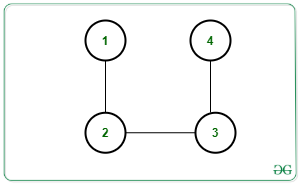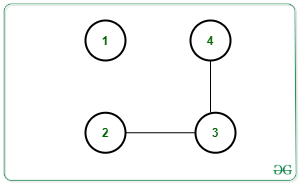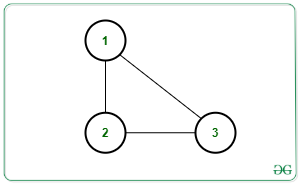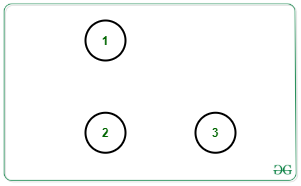Skip to content
Related Articles
Maximum number of edges to be removed to contain exactly K connected components in the Graph
• Difficulty Level : Medium
• Last Updated : 04 Feb, 2021

Given an undirected graph G with N nodes, M edges, and an integer K, the task is to find the maximum count of edges that can be removed such that there remains exactly K connected components after the removal of edges. If the graph cannot contain K connect components, print -1.

Examples:

Input: N = 4, M = 3, K = 2, Edges[][] = {{1, 2}, {2, 3}, {3, 4}}Output: 1
Explanation:
One possible way is to remove edge [1, 2]. Then there will be 2 connect components as shown below:Input: N = 3, M = 3, K = 3, Edges[][] = {{1, 2}, {2, 3}, {3, 1}}Output: 3
Explanation: All edges can be removed to make 3 connected components as shown below:Approach: To solve the given problem, count the number of connected components present in the given graph. Let the count be C. Observe that if C is greater than K then no possible edge removal can generate K connected components as the number of connected components will only increase. Otherwise, the answer will always exist.

Following observations need to be made in order to solve the problem:

• Suppose C1, C2, …, Cc, are the number of node in each connected component. Then, each component must have edges as C1 – 1, C2 – 1, …, C-1 after edges are removed. Therefore,

C1 – 1 + C2 – 1 + … + Cc – 1 = C1 + C2 + … + Cc – C = N – C, where N is the number of nodes.

• The above condition will give us the C connected components by removing M – (N – C) edges as N – C edges are needed to make C components. To get K components, (K – C) more edges must be removed.
• Hence, the total count of edges to be removed is given by:

M – (N – C) + (K – C) = M – N + K

Follow the steps below to solve the problem:

1. Count the number of connected components present in the given graph. Let the count be C.
2. If C is greater than K, print -1.
3. Else print M – N + K where N is the number f nodes, M is the number of edges and K is the required number of connected components.

Below is the implementation of the above approach:

## C++

 `// C++ program for the above approach``#include ``using` `namespace` `std;` `class` `Graph``{``  ``public``:``    ``int` `V;``    ``map<``int``, vector<``int``>> adj;` `    ``Graph(``int``);``    ``void` `addEdge(``int``, ``int``);``    ``void` `DFS(``int``, vector<``bool``> &);``} * g;` `// Constructor``Graph::Graph(``int` `V)``{``  ` `  ``// No. of vertices``  ``this``->V = V;``  ` `  ``// Dictionary of lists``  ``for``(``int` `i = 1; i <= V; i++)``    ``adj[i] = vector<``int``>();``}` `// Function to add edge``// in the graph``void` `Graph::addEdge(``int` `v, ``int` `w)``{``  ``adj[v].push_back(w);``  ``adj[w].push_back(v);``}` `// Function to perform DFS``void` `Graph::DFS(``int` `s, vector<``bool``> &visited)``{``  ` `  ``// Create a stack for DFS``  ``stack<``int``> stack;` `  ``// Push the current source node``  ``stack.push(s);``  ``while` `(!stack.empty())``  ``{``    ` `    ``// Pop a vertex from stack``    ``// and print it``    ``s = stack.top();``    ``stack.pop();` `    ``// Traverse adjacent vertices``    ``// of the popped vertex s``    ``for``(``auto` `node : adj[s])``    ``{``      ``if` `(!visited[node])``      ``{``        ` `        ``// If adjacent is unvisited,``        ``// push it to the stack``        ``visited[node] = ``true``;``        ``stack.push(node);``      ``}``    ``}``  ``}``}` `// Function to return the count``// edges removed``void` `countRemovedEdges(``int` `N, ``int` `M, ``int` `K)``{``  ``int` `C = 0;` `  ``// Initially mark all verices``  ``// as not visited``  ``vector<``bool``> visited(g->V + 1, ``false``);` `  ``for``(``int` `node = 1; node <= N; node++)``  ``{``    ` `    ``// If node is unvisited``    ``if` `(!visited[node])``    ``{``      ` `      ``// Increment Connected``      ``// component count by 1``      ``C = C + 1;` `      ``// Perform DFS Traversal``      ``g->DFS(node, visited);` `      ``// Print the result``      ``if` `(C <= K)``        ``cout << M - N + K << endl;``      ``else``        ``cout << -1 << endl;``    ``}``  ``}``}` `// Driver Code``int` `main(``int` `argc, ``char` `const` `*argv[])``{``  ``int` `N = 4, M = 3, K = 2;` `  ``// Create Graph``  ``g = ``new` `Graph(N);` `  ``// Given Edges``  ``g->addEdge(1, 2);``  ``g->addEdge(2, 3);``  ``g->addEdge(3, 4);` `  ``// Function Call``  ``countRemovedEdges(N, M, K);``}` `// This code is contributed by sanjeev2552`

## Java

 `// Java program to implement ``// the above approach``import` `java.util.*;``class` `GFG``{` `  ``static` `ArrayList> graph;` `  ``// Function to perform DFS``  ``static` `void` `DFS(``int` `s, ``boolean``[] visited)``  ``{` `    ``// Create a stack for DFS``    ``Stack stack = ``new` `Stack<>();` `    ``// Push the current source node``    ``stack.push(s);``    ``while` `(!stack.isEmpty())``    ``{` `      ``// Pop a vertex from stack``      ``// and print it``      ``s = stack.peek();``      ``stack.pop();` `      ``// Traverse adjacent vertices``      ``// of the popped vertex s``      ``for``(Integer node : graph.get(s))``      ``{``        ``if` `(!visited[node])``        ``{` `          ``// If adjacent is unvisited,``          ``// push it to the stack``          ``visited[node] = ``true``;``          ``stack.push(node);``        ``}``      ``}``    ``}``  ``}` `  ``// Function to return the count``  ``// edges removed``  ``static` `void` `countRemovedEdges(``int` `N, ``int` `M, ``int` `K)``  ``{``    ``int` `C = ``0``;` `    ``// Initially mark all verices``    ``// as not visited``    ``boolean``[] visited = ``new` `boolean``[N+``1``];` `    ``for``(``int` `node = ``1``; node <= N; node++)``    ``{` `      ``// If node is unvisited``      ``if` `(!visited[node])``      ``{` `        ``// Increment Connected``        ``// component count by 1``        ``C = C + ``1``;` `        ``// Perform DFS Traversal``        ``DFS(node, visited);` `        ``// Print the result``        ``if` `(C <= K)``          ``System.out.println(M - N + K);``        ``else``          ``System.out.println(-``1``);``      ``}``    ``}``  ``}` `  ``// Driver code``  ``public` `static` `void` `main (String[] args)``  ``{``    ``int` `N = ``4``, M = ``3``, K = ``2``;` `    ``// Create Graph``    ``graph = ``new` `ArrayList<>();` `    ``for``(``int` `i = ``0``; i <= N; i++)``      ``graph.add(``new` `ArrayList());` `    ``// Given Edges``    ``graph.get(``1``).add(``2``);``    ``graph.get(``2``).add(``3``);``    ``graph.get(``3``).add(``4``);` `    ``// Function Call``    ``countRemovedEdges(N, M, K);``  ``}``}` `// This code is contributed by offbeat.`

## Python3

 `# Python3 program for the above approach` `class` `Graph:` `    ``# Constructor``    ``def` `__init__(``self``, V):` `        ``# No. of vertices``        ``self``.V ``=` `V` `        ``# Dictionary of lists``        ``self``.adj ``=` `{i: [] ``for` `i ``in` `range``(``1``, V ``+` `1``)}` `    ``# Function to add edge``    ``# in the graph``    ``def` `addEdge(``self``, v, w):``        ``self``.adj[v].append(w)``        ``self``.adj[w].append(v)` `    ``# Function to perform DFS``    ``def` `DFS(``self``, s, visited):` `    ``# Create a stack for DFS``        ``stack ``=` `[]` `        ``# Push the current source node``        ``stack.append(s)``        ``while` `(``len``(stack)):` `            ``# Pop a vertex from stack``            ``# and print it``            ``s ``=` `stack[``-``1``]``            ``stack.pop()` `            ``# Traverse adjacent vertices``            ``# of the popped vertex s``            ``for` `node ``in` `self``.adj[s]:``                ``if` `(``not` `visited[node]):` `                    ``# If adjacent is unvisited,``                    ``# push it to the stack``                    ``visited[node] ``=` `True``                    ``stack.append(node)` `# Function to return the count``# edges removed``def` `countRemovedEdges(N, M, K):` `    ``C ``=` `0` `    ``# Initially mark all verices``    ``# as not visited``    ``visited ``=` `[``False` `for` `i ``in` `range``(g.V ``+` `1``)]` `    ``for` `node ``in` `range``(``1``, N ``+` `1``):` `        ``# If node is unvisited``        ``if` `(``not` `visited[node]):` `            ``# Increment Connected``            ``# component count by 1``            ``C ``=` `C ``+` `1` `            ``# Perform DFS Traversal``            ``g.DFS(node, visited)` `    ``# Print the result``    ``if` `C <``=` `K:``        ``print``(M ``-` `N ``+` `K)``    ``else``:``        ``print``(``-``1``)`  `# Driver Code` `N, M, K ``=` `4``, ``3``, ``2` `# Create Graph``g ``=` `Graph(N)` `# Given Edges``g.addEdge(``1``, ``2``)``g.addEdge(``2``, ``3``)``g.addEdge(``3``, ``4``)` `# Function Call``countRemovedEdges(N, M, K)`
Output:
`1`

Time Complexity: O(N + M)
Auxiliary Space: O(M + N)

Attention reader! Don’t stop learning now. Get hold of all the important DSA concepts with the DSA Self Paced Course at a student-friendly price and become industry ready.  To complete your preparation from learning a language to DS Algo and many more,  please refer Complete Interview Preparation Course.

In case you wish to attend live classes with industry experts, please refer Geeks Classes Live

My Personal Notes arrow_drop_up